# AQA A Level Maths: Statistics复习笔记3.2.1 Set Notation & Conditional Probability

### Set Notation

####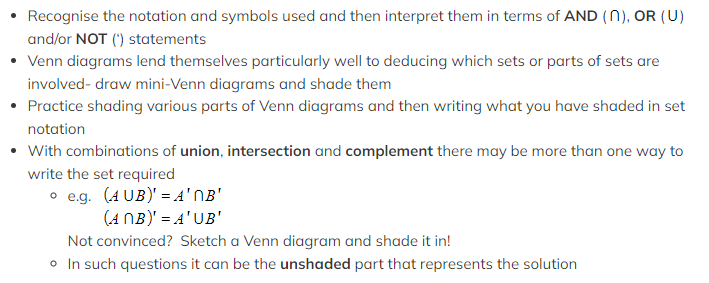Worked Example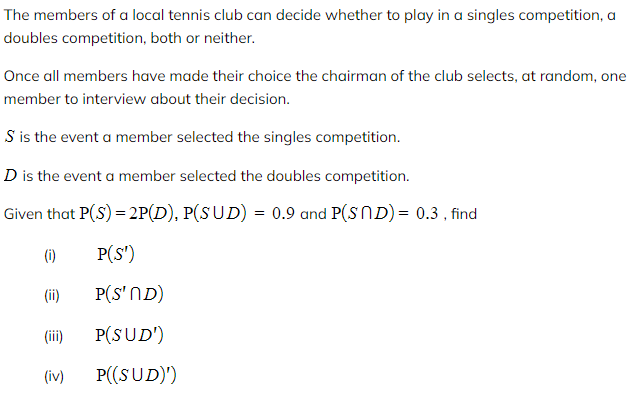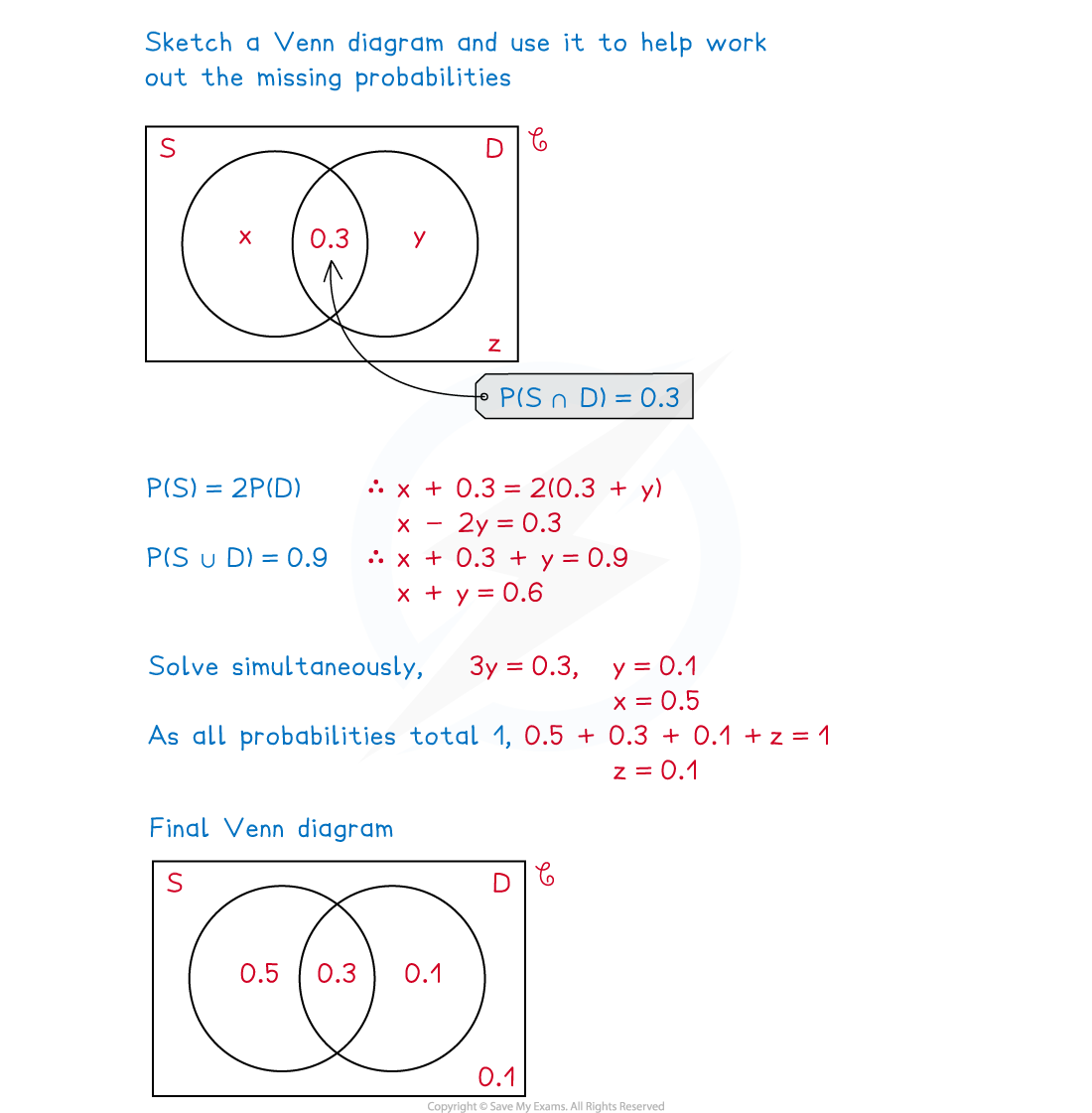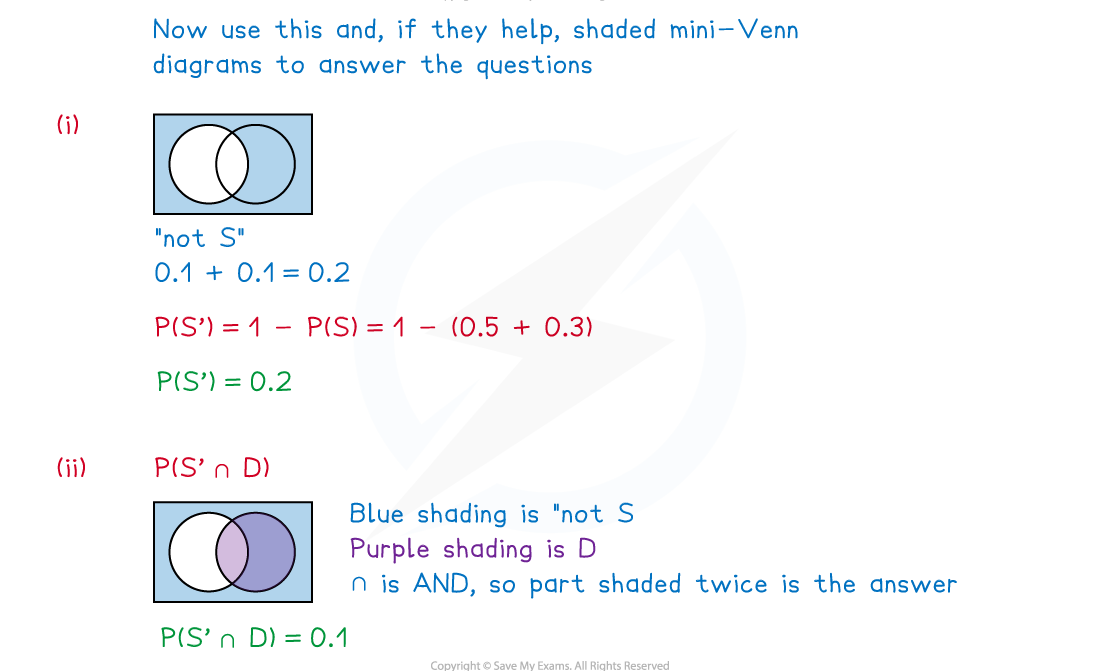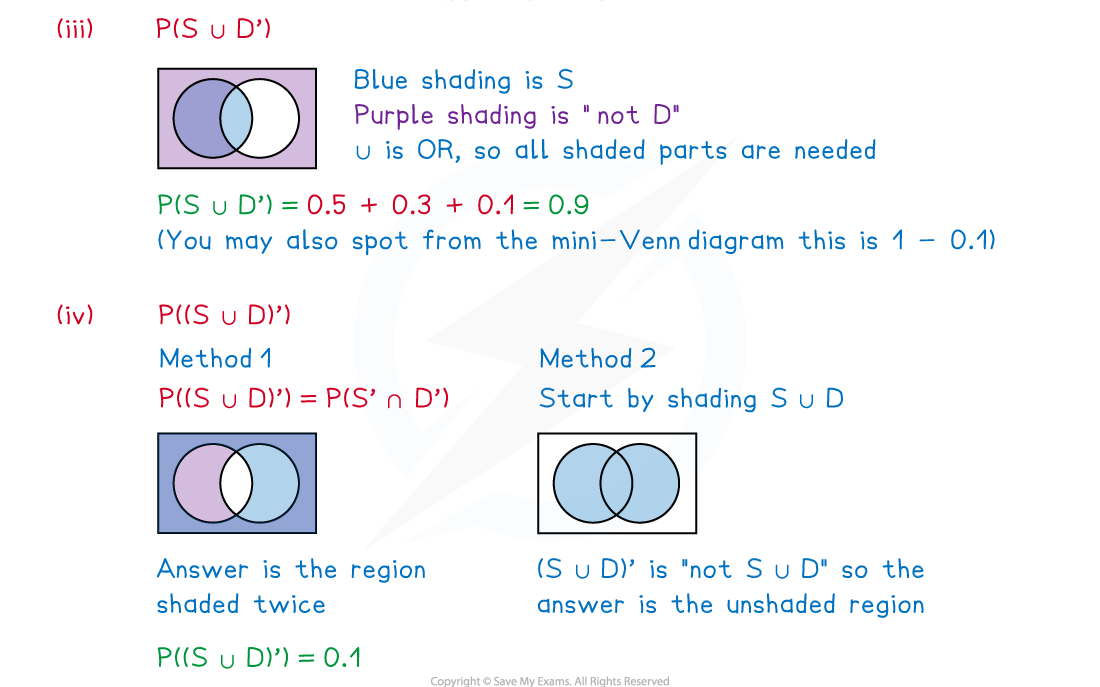### Conditional Probability

####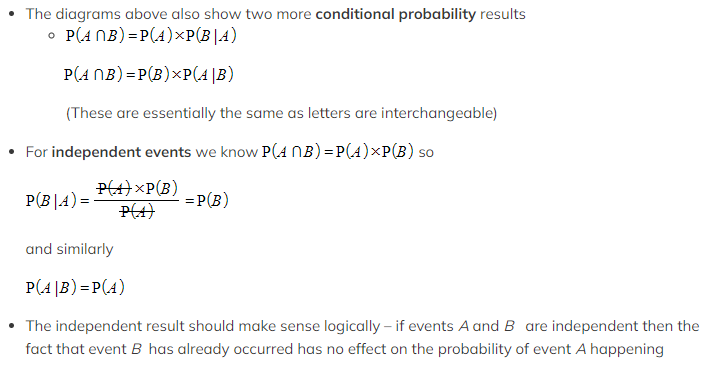Worked Example

The Venn diagram below illustrates the probabilities of three events, .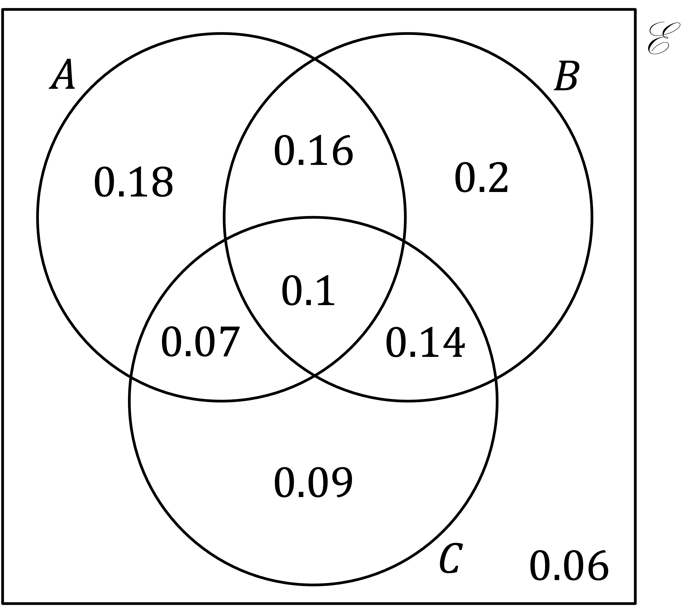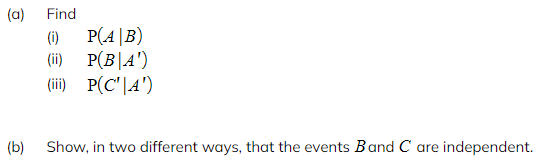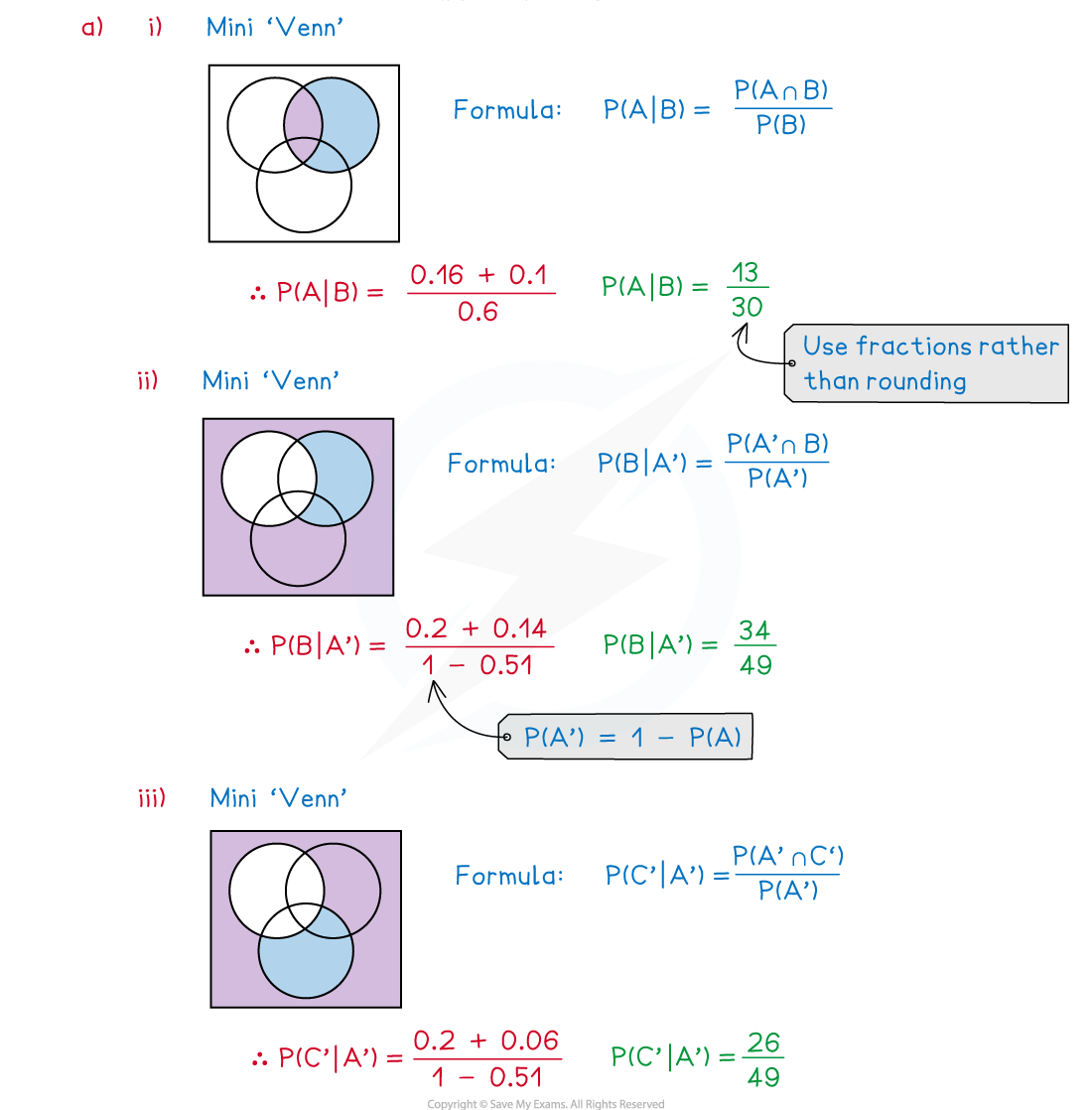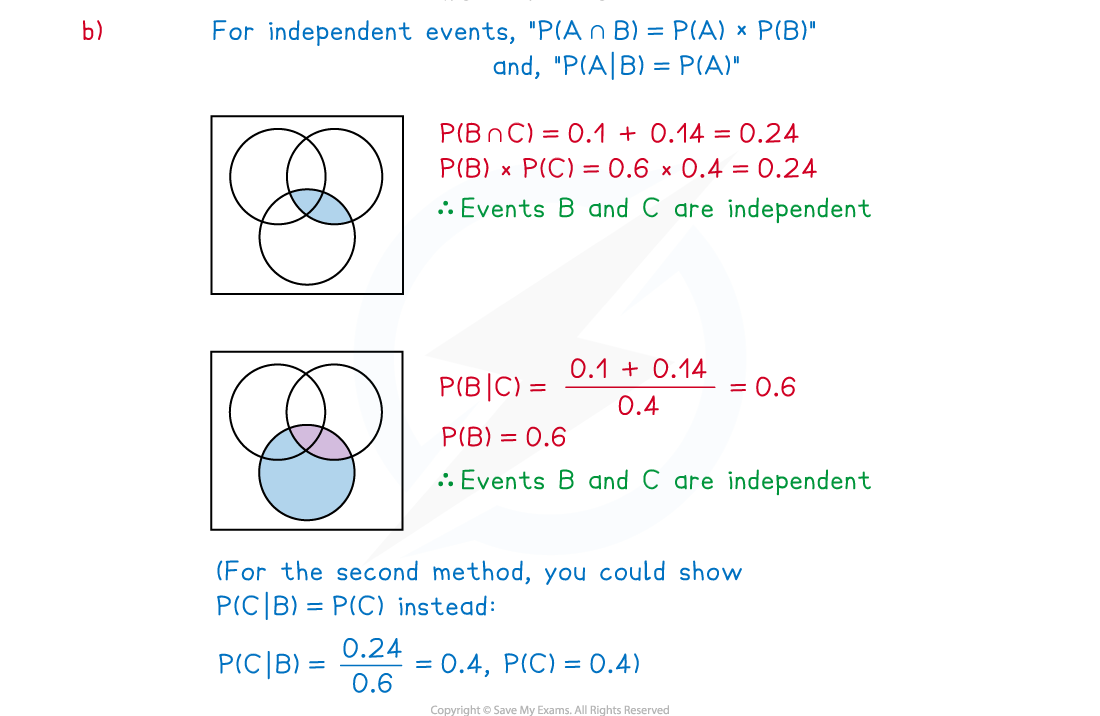### Two-Way Tables

#### Exam Tip

• Ensure any table – given or drawn - has a “Total” row and a “Total” column
• Do not confuse a two-way table with a sample space diagram – a two-way table does not necessarily display all outcomes from an experiment, just those (events) we are interested in